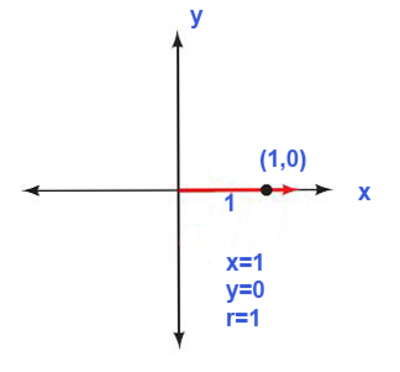## Trigonometry 7th Edition

To find out the value of $\sec 0^{\circ}$- We will consider $90^{\circ}$ in a position such that y=0. locate point (1.0) on the terminal side and use Definition I- Using the point (1,0) x = 1, y = 0 and r = 1 $\sec 0^{\circ} = \frac{r}{x}$ $\sec 0^{\circ} = \frac{1}{1}$ $\sec 0^{\circ}$ = 1